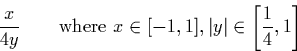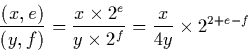Next: High-Level Operations Up: Division Previous: Output negative digits

### Extending division to the whole real line

Extending the division algorithm to work on the whole real line is relatively trivial. We now have an algorithm which will computewith the precondition that y is of the form (1::0::y') or (1::1::y'). We can now implement the full division algorithm using the following observation:Appendix B gives the Haskell implementation of the entire division algorithm. The structure closely follows the approach taken in describing the algorithm here, and gives a succinct recursive definition for the algorithm.

Martin Escardo
5/11/2000